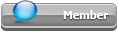## Featured Articles

Check out the latest featured articles.## File Library0# Calculation Of Standard Gas Volume Well Operations

z-factor gas volume oil well nitrogen

3 replies to this topic
|

### #1Daniel89

Daniel89

Brand New Member

•• Members
•• 8 posts

Posted 12 November 2018 - 08:05 PM

Hi!

I need help in how to calculate standard volume of gas needed to pressurize an oilwell from X pressure to Y pressure.

I have made macros to calculate Z- factor and density of the gas by the Soavey Redlich Kwong EOS. I know the temperature gradient of the geology so I know the temperature pretty exact down the wellbore, this is also confirmed by the Downhole pressure and temperature gauge.

The calculated density of the gas is pretty spot on based on pressure measurements from the mentioned Downhole pressure gauge which verifies the calculated hydrostatic pressure exerted by the gas column. The density is calculated for 1000 points down the wellbore, i.e a good representation of the "average" Z- factor/density exists.

The well is initially filled with a brine-based completion fluid, then the well is displaced to pure nitrogen gas by circulating the fluids out of the well by the annular cavity, the wellbore is isolated from the reservoir by a deep set plug which is pressure tested. and the annulus valve is closed and pressuretested i.e:

- There is a certain limited free-gas volume which can be calculated with high precision

- The temperature is known through the wellbore, and the pressure and density is calculated

-The gas stays in the well for a long time, i.e. the gas temperature will be close to the geology temperature, before the gas is bled off through the flare line.

So far all good, my calculations yields high precision until I try to calculate the standard volume of gas needed for the operation.

My macro for calculating standard volume of nitrogen needed:

Function Standard_Gas_Volume(P_initial_Bar, T1_Celsius, V_m3, P_final_Bar, T2_Celsius)

'to calculate a standard m³ of gas that needs to be bled off or added to at pressure containing vessel

'to pressurise or bleed off pressure to P_Final_Bar

'Z values calculated by Soavey Redlich Kwong EOS, output in sm³

'P1_Bar&P2_Bar in Bar

'T1_Celsius&T2_Celsius in Celsius

'V_m3 Volume of the pressure containing vessel in m³

T1_K = Kelvin(T1_Celsius) 'Converts Celsius to Kelvin

T2_K = Kelvin(T2_Celsius) 'Converts Celsius to Kelvin

P1_P = Pressure_Pa(P_initial_Bar) 'Converts Bar to Pascal overpressure

P2_P = Pressure_Pa(P_final_Bar) 'Converts Bar to Pascal overpressure

Z_1 = SRK_N2_Z(P_initial_Bar, T1_Celsius) 'Z factor 1

Z_2 = SRK_N2_Z(P_final_Bar, T2_Celsius) 'Z factor 2

Z_atm = SRK_N2_Z(atm_Bar, T2_Celsius) ' Z factor atmospheric

If P2_P & P1_P = 0 Then

Standard_Gas_Volume = 0

ElseIf P2_P = 0 Then

Standard_Gas_Volume = ((P1_P) / atm_Pa) / (Z_1 / Z_atm) * (T2_K / T1_K) * V_m3

Else

Standard_Gas_Volume = ((Abs(P1_P - P2_P)) / atm_Pa) / ((Z_2 + Z_1) / 2) * (T2_K / T1_K) * V_m3

End If

End Function

Question: I don't seem to get this right, Should I use the average Z-factor of the whole wellbore for both pressures, or the Z- factor of the gas at the "top of the well? I don't seem to get this right.

All help is certainly much appreciated.

Best Regards, Daniel

### #2Bobby Strain

Bobby Strain

Gold Member

•• Members
•• 2,901 posts

Posted 12 November 2018 - 10:41 PM

You must already know the density down the bore. Otherwise you couldn't calculate the pressure profile. So, use it.

Bobby

### #3shan

shan

Gold Member

•• ChE Plus Subscriber
•• 692 posts

Posted 13 November 2018 - 03:44 PM

You may calculate the amount of moles (n) in each of wellbore section with n = PV/(ZRT) with the average P, V and T values in the consider section and multiply the sum of n with standard gas volume to get the total volume in the standard conditions.

### #4Daniel89

Daniel89

Brand New Member

•• Members
•• 8 posts

Posted 20 November 2018 - 02:50 AM

Thank you for helpful inputI took the mass approach, and calculated the mass of the gas in the wellbore based on the wellbore free-gas volume and the average density based on 1000 datapoints down the wellbore.

The macro calculates the difference in mass in situation x and y. Then I used SRK to calculate the mass of 1m3 of gas at STP conditions and divided the mass difference in the wellbore with the result.

I believe this is an apropriate method, agree?

Best regards, Daniel